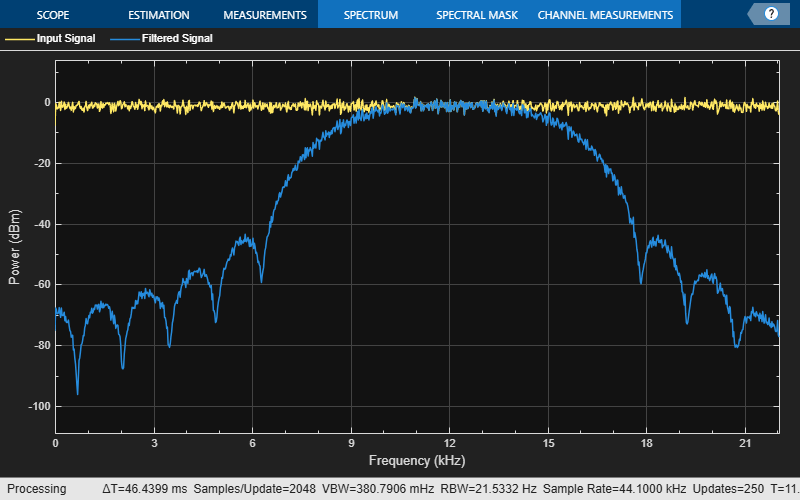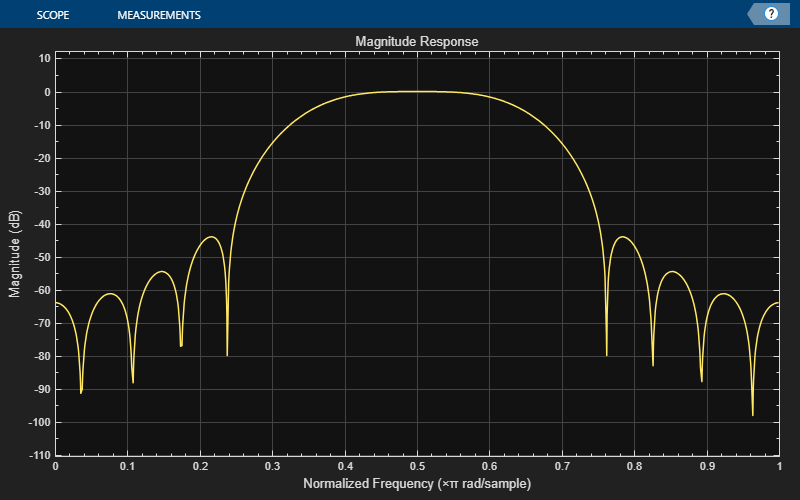# designBandpassFIR

Design and implement bandpass FIR filter

Since R2023b

## Syntax

``B = designBandpassFIR``
``B = designBandpassFIR(Name=Value)``

## Description

example

````B = designBandpassFIR` designs a bandpass FIR filter with the filter order of 100, center frequency of 0.5, bandwidth of 0.1, and a Hamming window. B is a vector of filter coefficients of length 101.The System object™ argument is `false` by default. To implement the filter, assign the filter coefficients in `B` to a `dsp.FIRFilter` object.```

example

````B = designBandpassFIR(Name=Value)` specifies options using one or more name-value arguments.For example, ```B = designBandpassFIR(FilterOrder=30,CenterFrequency=0.4,Window="hann",SystemObject=true)``` designs a bandpass FIR filter with the filter order of 30, center frequency of 0.4, and a Hann window. As the `SystemObject` argument is `true`, the function designs and implements the bandpass FIR filter. `B` is a `dsp.FIRFilter` System object in this case. When you specify only a partial list of filter parameters, the function designs the filter by setting the other design parameters to their default values.```

## Examples

collapse all

Create a `dsp.FIRFilter` object, and set the `NumeratorSource` property to `'Input port'` so that you can vary the coefficients of the FIR filter through the input port during simulation.

`firFilt = dsp.FIRFilter(NumeratorSource="Input port")`
```firFilt = dsp.FIRFilter with properties: Structure: 'Direct form' NumeratorSource: 'Input port' InitialConditions: 0 Use get to show all properties ```

Create a `spectrumAnalyzer` object to visualize the spectra of the input and output signals.

```spectrumScope = spectrumAnalyzer(SampleRate=44100,PlotAsTwoSidedSpectrum=false,... ChannelNames=["Input Signal","Filtered Signal"]);```

Create a `dsp.DynamicFilterVisualizer` object to visualize the magnitude response of the varying filter.

`filterViz = dsp.DynamicFilterVisualizer(NormalizedFrequency=true);`

Stream in random data and filter the signal using the `dsp.FIRFilter` object. Use the `designBandpassFIR` function to design the filter coefficients. By default, this function returns a vector of FIR filter coefficients. Assign these coefficients to the `dsp.FIRFilter` object.

Vary the center frequency of the filter during simulation. The `designBandpassFIR` function redesigns the coefficients based on the updated filter specifications. Pass these updated coefficients to the FIR filter. Visualize the spectra of the input and filtered signals using the spectrum analyzer.

```Fc = 0.5; for idx = 1:500 num = designBandpassFIR(FilterOrder=30,CenterFrequency=Fc,Bandwidth=0.3,Window="hann"); x = randn(1024,1); y = firFilt(x,num); spectrumScope(x,y); filterViz(num); Fc = Fc + 0.0001; end```Design and implement a bandpass FIR filter object using the `designBandpassFIR` function. The function returns a `dsp.FIRFilter` object when you set the `SystemObject` argument to `true`.

```firFilt = designBandpassFIR(FilterOrder=30,CenterFrequency=0.5,Bandwidth=0.3,Window="hann",... SystemObject=true)```
```firFilt = dsp.FIRFilter with properties: Structure: 'Direct form' NumeratorSource: 'Property' Numerator: [0 -1.5217e-04 3.2176e-19 -0.0030 2.0714e-17 0.0158 -1.1893e-17 -0.0208 3.3379e-18 -0.0213 2.0490e-17 0.1252 -3.4516e-17 -0.2442 1.7349e-17 0.2973 1.7349e-17 -0.2442 -3.4516e-17 0.1252 2.0490e-17 -0.0213 ... ] (1x31 double) InitialConditions: 0 Use get to show all properties ```

Create a `dsp.DynamicFilterVisualizer` object to visualize the magnitude response of the varying filter.

```filterViz = dsp.DynamicFilterVisualizer(NormalizedFrequency=true); filterViz(firFilt)```Create a `spectrumAnalyzer` object to visualize the spectra of the input and output signals.

```spectrumScope = spectrumAnalyzer(SampleRate=44100,PlotAsTwoSidedSpectrum=false,... ChannelNames=["Input Signal","Filtered Signal"]);```

Stream in random data and filter the signal using the `dsp.FIRFilter` object. Visualize the spectra of the input and filtered signals using the spectrum analyzer.

```for idx = 1:500 x = randn(1024,1); y = firFilt(x); spectrumScope(x,y); end```## Input Arguments

collapse all

### Name-Value Arguments

Specify optional pairs of arguments as `Name1=Value1,...,NameN=ValueN`, where `Name` is the argument name and `Value` is the corresponding value. Name-value arguments must appear after other arguments, but the order of the pairs does not matter.

Example: `designBandpassFIR(FilterOrder=30,CenterFrequency=0.3,Bandwidth=0.2)`

Order of the bandpass FIR filter, N, specified as an even nonnegative integer.

Data Types: `single` | `double` | `int8` | `int16` | `int32` | `int64` | `uint8` | `uint16` | `uint32` | `uint64`

Center frequency of the bandpass FIR filter, Fc, specified as a normalized scalar in the range (`0`,`1`].

Data Types: `single` | `double`

Bandwidth of the bandpass FIR filter, BW, specified as a normalized scalar in the range (`0`,`1`].

Data Types: `single` | `double`

Window design method, specified as one of these options:

• `"hamming"`

• `"hann"`

• `"blackman"`

• `"blackman-harris"`

• `"chebyshev"` –– The default sidelobe attenuation is 60 dB.

• `"kaiser"`

• `"custom"` –– Specify the custom window vector in the `CustomWindow` argument.

Data Types: `char` | `string`

Custom window vector, specified as a vector of length `FilterOrder` + 1.

Data Types: `single` | `double`

Option to create System object, specified as one of these:

• `false` –– The function returns a vector of FIR filter coefficients.

• `true` –– The function returns a `dsp.FIRFilter` object.

Data Types: `logical`

Option to print the entire function call in MATLAB, specified as one of these:

• `false` –– The function does not print the function call.

• `true` –– The function prints the entire function call including the default values of the `Name=Value` arguments that you did not specify when calling the function.

Use this argument to view all the values used by the function to design and implement the filter.

Data Types: `logical`

## Output Arguments

collapse all

Bandpass FIR filter coefficients or filter object, returned as one of these:

Data Types: `single` | `double`

## Version History

Introduced in R2023b